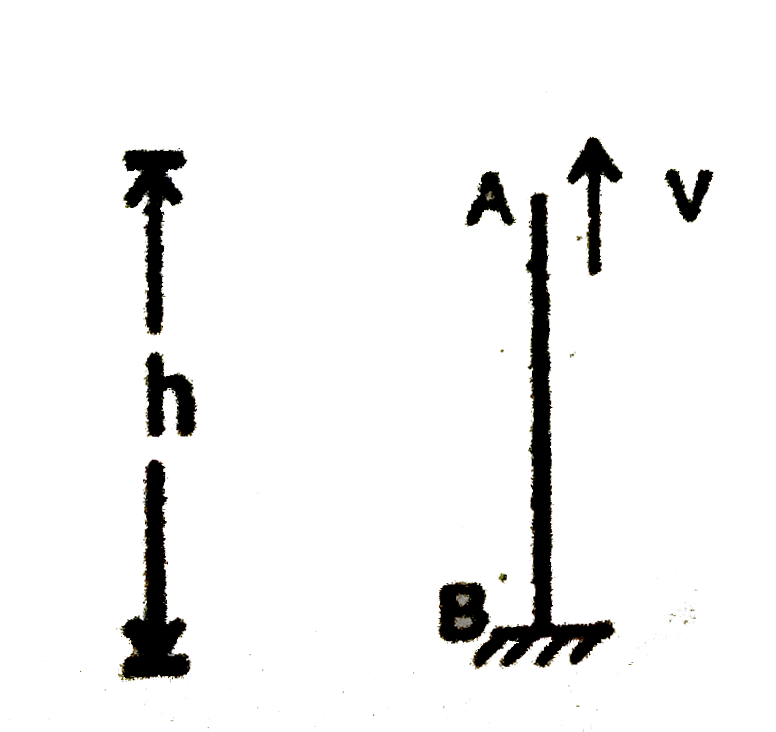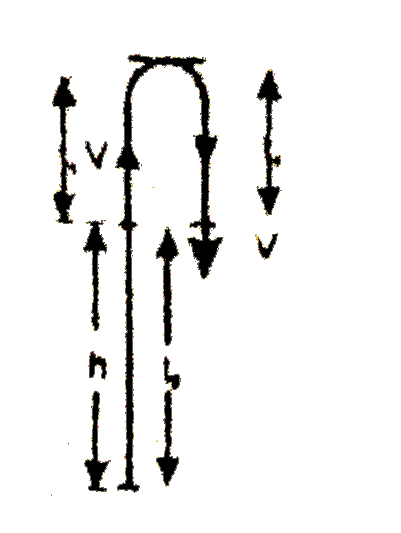# A ball is thrown vertically upwards from the top of tower of height h with velocity v . The ball strikes the ground after time.

1.6k views
in Physics
closed
A ball is thrown vertically upwards from the top of tower of height h with velocity v . The ball strikes the ground after time.
A. v/g[1+sqrt(1+(2gh)/(v^(2)))]
B. v/g[1+sqrt(1-(2gh)/(v^(2)))]
C. (v)/(g)(1+(2gh)/(v^(2)))^(1//2)
D. (v)/(g)(1-(2gh)/(v^(2)))^(1//2)

by (84.1k points)
selectedFor AB " " AB
s=ut+1/2 at^(2).
-h=vt-g/2 t^(2)
g/2 t^(2)-vt-h=0
t=(vpmsqrt(v^(2)+4xxg/2xxh))/(2xxg/2)
t=(vpmsqrt(v^(2)+2gh))/g
t=v/g[1+sqrt(1+(2gh)/(v^(2)))], t=v/g[1-sqrt(1+(2gh)/(v^(2)))] [as time cannot be negative so we neglect it we neglect]
:. t=v/g[1+sqrt(1+(2gh)/(v^(2)))]
AliterLet t_(1) be the time taken by ball from top of tower to the highest point then it will taken again t_(1) time to return back to the top of tower Let t_(2) be the time taken be ball from top of tower of the ground.
For t_(1): From equation
V=u-gt i.e. 0=V-gt_(1) or, t_(1)=V//g
For t_(2): from equation
h=ut+1/2 g t^(2) h=V t^(2)+1/2 g t_(2)^(2): or , g t_(2)^(2)+2Vt_(2)-2h=0, or t_(2)=(-2v pm sqrt(4V^(2)+8gh))/(2g)
Taking (+) sign only (as we are interested in time projection i.e. t=0) t_(2)=(-Vpmsqrt(V^(2)+2gh))/g
Note that, -ve time indicate time before the projection
Hence, the time after which the ball strikes ground T=2t_(1)+t_(2) rArr T=(2v)/g+(-V+sqrt(V^(2)+2gh))/g
T=(V+sqrt(V^(2)+2gh))/grArr T=V/g[1+sqrt(1+(2gh)/(V^(2)))]